# Limit set of a trajectory

(diff) ← Older revision | Latest revision (diff) | Newer revision → (diff)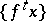of a dynamical system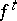The set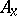of all-limit points (the-limit set) or the set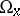of all-limit points (the-limit set) of this trajectory (cf. Limit point of a trajectory). The-limit set (-limit set) of a trajectoryof a system (or, in other notation,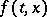, cf. ) is the same as the-limit set (respectively,-limit set) of the trajectory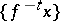of the dynamical system(the system with reversed time). Therefore the properties of-limit sets are similar to those of-limit sets.

The setis a closed invariant set. If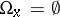, then the trajectoryis called divergent in the positive direction; if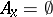, divergent in the negative direction; if, the trajectory is called divergent. If, thenis called positively Poisson stable; if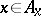, negatively Poisson stable; and if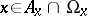, thenis called Poisson stable. If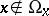and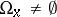, thenis called positively asymptotic; ifand, the pointis called negatively asymptotic.

Ifis a positively Lagrange-stable point (cf. Lagrange stability), thenis a non-empty connected set,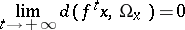(where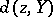is the distance from a pointto a set) and there is a recurrent point (trajectory) in. Ifis a fixed point, then. Ifis a periodic point, then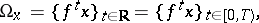whereis the period. Ifis not a fixed point and not a periodic point, and if the underlying metric space of the dynamical system under consideration is complete, then the points innot on the trajectoryare everywhere-dense in.

If a dynamical system in the plane is given by an autonomous system of differential equations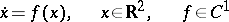(with a smooth vector field),is positively Lagrange stable but not periodic, anddoes not vanish on(i.e.does not contain fixed points), thenis a cycle, i.e. a closed curve (the trajectory of a periodic point), while the trajectorywinds, spiral-wise, around this cycle as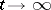. For dynamical systems in,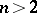, or on a two-dimensional surface, e.g. a torus, the-limit sets can have a different structure. E.g., for an irrational winding on a torus (the system,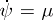, where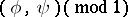are cyclic coordinates on the torusandis an irrational number) the setcoincides, for every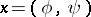, with the torus.

How to Cite This Entry:
Limit set of a trajectory. Encyclopedia of Mathematics. URL: http://encyclopediaofmath.org/index.php?title=Limit_set_of_a_trajectory&oldid=14411
This article was adapted from an original article by V.M. Millionshchikov (originator), which appeared in Encyclopedia of Mathematics - ISBN 1402006098. See original article